# How to create and use dynamic named range in Excel

In this tutorial, you will learn how to create a dynamic named range in Excel and how to use it in formulas to have new data included in calculations automatically.

In last week's tutorial, we looked at different ways to define a static named range in Excel. A static name always refers to the same cells, meaning you would have to update the range reference manually whenever you add new or remove existing data.

If you are working with a continuously changing data set, you may want to make your named range dynamic so that it automatically expands to accommodate newly added entries or contracts to exclude removed data. Further on in this tutorial, you will find detailed step-by-step guidance on how to do this.

## How to create a dynamic named range in Excel

For starters, let's build a dynamic named range consisting of a single column and a variable number of rows. To have it done, perform these steps:

1. On the Formula tab, in the Defined Names group, click Define Name. Or, press Ctrl + F3 to open the Excel Name Manger, and click the New… button.
2. Either way, the New Name dialogue box will open, where you specify the following details:
• In the Name box, type the name for your dynamic range.
• In the Scope dropdown, set the name's scope. Workbook (default) is recommended in most cases.
• In the Refers to box, enter either OFFSET COUNTA or INDEX COUNTA formula.
3. Click OK. Done!

In the following screenshot, we define a dynamic named range items that accommodates all cells with data in column A, except for the header row: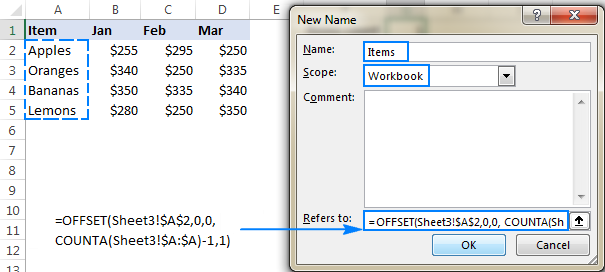### OFFSET formula to define an Excel dynamic named range

The generic formula to make a dynamic named range in Excel is as follows:

OFFSET(first_cell, 0, 0, COUNTA(column), 1)

Where:

• first_cell - the first item to be included in the named range, for example \$A\$2.
• column - an absolute reference to the column like \$A:\$A.

At the core of this formula, you use the COUNTA function to get the number of non-blank cells in the column of interest. That number goes directly to the height argument of the OFFSET(reference, rows, cols, [height], [width]) function telling it how many rows to return.

Beyond that, it's an ordinary Offset formula, where:

• reference is the starting point from which you base the offset (first_cell).
• rows and cols are both 0, since there are no columns or rows to offset.
• width is equal to 1 column.

For example, to build a dynamic named range for column A in Sheet3, beginning in cell A2, we use this formula:

`=OFFSET(Sheet3!\$A\$2, 0, 0, COUNTA(Sheet3!\$A:\$A), 1)`

Note. If you are defining a dynamic range in the current worksheet, you do not need to include the sheet name in the references, Excel will do it for you automatically. If you are building a range for some other sheet, prefix the cell or range reference with the sheet's name followed by the exclamation point (like in the formula example above).

### INDEX formula to make a dynamic named range in Excel

Another way to create an Excel dynamic range is using COUNTA in combination with the INDEX function.

first_cell:INDEX(column,COUNTA(column))

This formula consists of two parts:

• On the left side of the range operator (:), you put the hard-coded starting reference like \$A\$2.
• On the right side, you use the INDEX(array, row_num, [column_num]) function to figure out the ending reference. Here, you supply the entire column A for the array and use COUNTA to get the row number (i.e. the number of non-entry cells in column A).

For our sample dataset (please see the screenshot above), the formula goes as follows:

`=\$A\$2:INDEX(\$A:\$A, COUNTA(\$A:\$A))`

Since there are 5 non-blank cells in column A, including a column header, COUNTA returns 5. Consequently, INDEX returns \$A\$5, which is the last used cell in column A (usually an Index formula returns a value, but the reference operator forces it to return a reference). And because we have set \$A\$2 as the starting point, the final result of the formula is the range \$A\$2:\$A\$5.

To test the newly created dynamic range, you can have COUNTA fetch the items count:

`=COUNTA(Items)`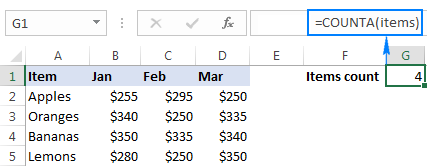If all done properly, the result of the formula will change once you add or remove items to/from the list: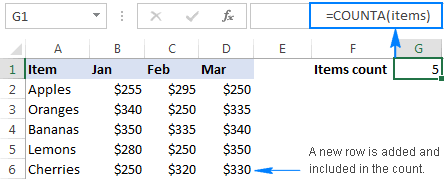Note. The two formulas discussed above produce the same result, however there is a difference in performance you should be aware of. OFFSET is a volatile function that recalculates with every change to a sheet. On powerful modern machines and reasonably sized data sets, this should not be a problem. On low-capacity machines and large data sets, this may slow down your Excel. In that case, you'd better use the INDEX formula to create a dynamic named range.

## How to make two-dimensional dynamic range in Excel

To build a two-dimensional named range, where not only the number of rows but also the number of columns is dynamic, use the following modification of the INDEX COUNTA formula:

first_cell:INDEX(\$1:\$1048576, COUNTA(first_column), COUNTA(first_row)))

In this formula, you have two COUNTA functions to get the last non-empty row and last non-empty column (row_num and column_num arguments of the INDEX function, respectively). In the array argument, you feed the entire worksheet (1048576 rows in Excel 2016 - 2007; 65535 rows in Excel 2003 and lower).

And now, let's define one more dynamic range for our data set: the range named sales that includes sales figures for 3 months (Jan to Mar) and adjusts automatically as you add new items (rows) or months (columns) to the table.

With the sales data beginning in column B, row 2, the formula takes the following shape:

`=\$B\$2:INDEX(\$1:\$1048576,COUNTA(\$B:\$B),COUNTA(\$2:\$2))`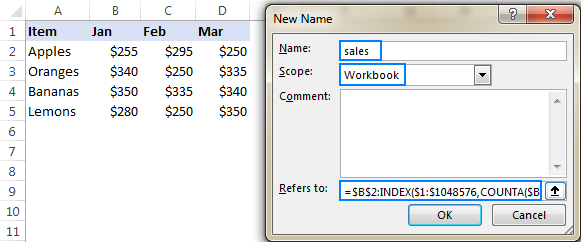To make sure your dynamic range works as it is supposed to, enter the following formulas somewhere on the sheet:

`=SUM(sales)`

`=SUM(B2:D5)`

As you can see in the screenshot bellow, both formulas return the same total. The difference reveals itself in the moment you add new entries to the table: the first formula (with the dynamic named range) will update automatically, whereas the second one will have to be updated manually with each change. That makes a huge difference, uh?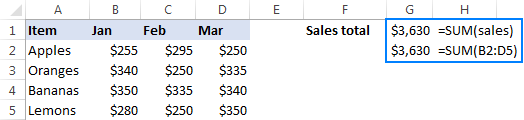## How to use dynamic named ranges in Excel formulas

In the previous sections of this tutorial, you have already seen a couple of simple formulas that use dynamic ranges. Now, let's try to come up with something more meaningful that shows the real value of an Excel dynamic named range.

For this example, we are going to take the classic INDEX MATCH formula that performs Vlookup in Excel:

INDEX (return_range, MATCH (lookup_valuelookup_range, 0))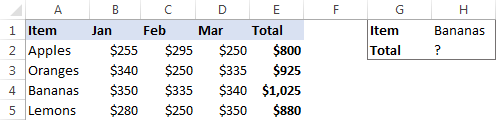…and see how we can make the formula even more powerful with the use of dynamic named ranges.

As shown in the screenshot above, we are attempting to build a dashboard, where the user enters an item name in H1 and gets the total sales for that item in H2. Our sample table created for demonstration purposes contains only 4 items, but in your real-life sheets there can be hundreds and even thousands of rows. Furthermore, new items can be added on a daily basis, so using references is not an option, because you'd have to update the formula over and over again. I'm too lazy for that! :)

To force the formula to expand automatically, we are going to define 3 names: 2 dynamic ranges, and 1 static named cell:

Lookup_range: =\$A\$2:INDEX(\$A:\$A, COUNTA(\$A:\$A))

Return_range: =\$E\$2:INDEX(\$E:\$E, COUNTA(\$E:\$E))

Lookup_value: =\$H\$1

Note. Excel will add the name of the current sheet to all references, so before creating the names be sure to open the sheet with your source data.

Now, start typing the formula in H1. When it comes to the first argument, type a few characters of the name you want to use, and Excel will show all available matching names. Double-click the appropriate name, and Excel will insert it in the formula right away: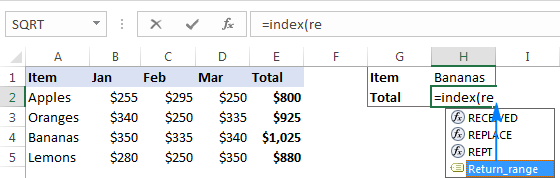The completed formula looks as follows:

`=INDEX(Return_range, MATCH(Lookup_value, Lookup_range, 0))`

And works perfectly!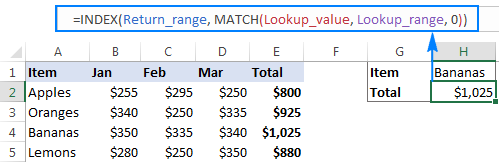As soon as you add new records to the table, they will be included in your calculations at once, without you having to make a single change to the formula! And if you ever need to port the formula to another Excel file, simply create the same names in the destination workbook, copy/paste the formula, and get it working immediately.

Tip. Apart from making formulas more durable, dynamic ranges come in handy for creating dynamic dropdown lists.

This is how you create and use dynamic named ranges in Excel. To have a closer look the formulas discussed in this tutorial, you are welcome to download our sample Excel Dynamic Named Range Workbook. I thank you for reading and hope to see you on our blog next week!

## You may also be interested in

### 14 responses to "How to create and use dynamic named range in Excel"

1.Rodney says:

How do I create a Dynamic Named Range with worksheets instead of rows or columns?

I have a workbook that I add a new worksheet to every week and I want to track my vacation time over the course of the calendar year.

2.Qudsia says:

Thank you

3.EDWIN says:

THANK YOU FOR ENLIGHTENING ME
AM SO DAMN GRATEFUL FOR THIS WRITE UP

4.Charles says:

Hi
How do you create a Dynamic named range (with INDEX) but without the sheet name automatically added after the Define Name editor is closed?
Tnks

5.ishan says:

Hi,

This index formula method for creating dynamic named range is not working.

Sheet3!\$H\$2:INDEX(Sheet3!\$H:\$H,COUNTA(Sheet3!\$H:\$H))

Using evaluate formula, this shows to result to \$H\$2:\$H\$6. But post that it acts as an array formula. Its finally returning value either H2/H3/H4/H5/H6 based on the cell.
What i mean to say is, it is not giving a range. Hence, this seems wrong.

6.LauraGayle says:

I'm trying to create a dynamic range showing client names only if their status is Active in another column. Can someone help me with the formula for this?

7.Flemming says:

An easy way to do it if you need a range is to use indirect in the name.. eg example for A2:G10
There is 20 rows in coloumn A

=Indirect(Sheet_name!A2:G"&CountA(A:A))

since indirect "translate" the expression - so it reads the name to be refered to this
Sheet_name!A2:G10

•Flemming says:

Correction - There is 20 rows in coloumn A - should ofcourse be 10 rows ;)

8.Mort says:

I have a simple table: 7 columns, 4 rows. Can I create a formula just adding Hrs of bananas?

Jan 1 Jan 2 Jan 3
Hrs Cases Hrs Cases Hrs Cases
Bananas 1 10 3 30 0 0
Apples 5 20 1 4 3 12

•Mort says:

_______|___Hrs_|_Cases_|_Hrs_|_Cases_|_Hrs_|_Cases
Bananas|____1__|___10__|__3__|___30___|_0__|___0__
Apples_|____5__|___20__|__1__|___04___|_3__|___12_

No sure if this will help to understand the table...

9.Aksel Alvarez says:

Hi! I'm a newbie in Excel, so there's a risk my question is kind os obvious. I'm trying to connect a Pivot Table in Excel to a Word File, for this, I have to edit the Name of my range in Excel and Word so the Word table automatically updates the range it is reporting. The problem I have is Excel says he doesn't understand my formula, it looks like this:

='DE40'!\$B\$4:\$B\$4:INDEX('DE40'!\$1:\$1048576,COUNTA('DE40'!\$B:\$B),+'DE40'!\$4:\$4))

Where DE40 is the Sheet/Pivot Table I'm trying to refer. Any ideas?

10.Justin Griffith says:

Hello, I am painfully new to working with Excel, macros, and the like. I have recorded a macro, but right now it will only work on the cell range that I originally recorded the macro on. Right now the range reads as =Range("A2:A724"), but I need the bottom part of the range (A724) to be dependent on column B. Meaning, if column B has data going down to cell B1021, then I want my range in column A to automatically look like =Range("A2:A1021"). I would appreciate any help. Thanks!

11.Saurabh says:

hi there.. hope someone could help me. I want to create a name in my file which is getting values from a single column table. I want to exclude one specific value and don't want it to be part of the name range. Not sure how can I do that? Column data is:
12.Ofer says: